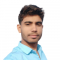## Half Subtractor Using NAND GatesUpdated on 26-Dec-2022 18:14:43

5K+ Views

In digital electronics, a subtractor is a combinational logic circuit that performs the subtraction of two binary numbers. However, the subtraction of binary number can be performed using adder circuits by taking 1’s or 2’s compliments. But, we may also realize a dedicate circuit to perform the subtraction of two binary numbers. In the subtraction of two binary numbers, each subtrahend bit of the number is subtracted from its corresponding significant minuend bit to form a difference bit. During the subtraction, if the minuend bit is smaller than the subtrahend bit, then a 1 is borrowed from the next position. ... Read More

## Half Adder with NAND GatesUpdated on 26-Dec-2022 18:08:59

12K+ Views